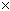SEARCH HOMEMath Central Quandaries & QueriesQuestion from janna, a student: After taking 4 quizes your average is 71 out of 100. what must your average on the next 5 quizes be to increase your average to an 81 out of 100?Hi Janna,

Suppose that S is the sum of your scores on the first 4 quizzes then S/4 = 71 so S = 471 = 284.

Suppose that the sum of your grades on the next 5 quizzes is T then the total of your grades on all 9 quizzes is S + T = 284 + T.

You want the average on all 9 quizzes to be 81 so (284 + T)/9 = 81.

Solve for T. What is your average on quizzes 5 to 9?

PennyMath Central is supported by the University of Regina and The Pacific Institute for the Mathematical Sciences.